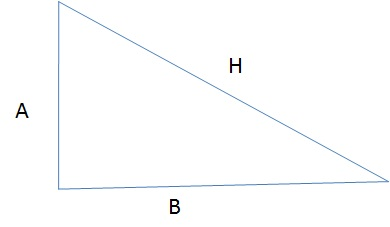# sqrt()

We can get square root of any number by using sqrt function, here are some examples.
``````select sqrt(16); // output is 4
select sqrt(20); // output is 4.47213595499958
``````
For negative number we will get NULL output
``select sqrt(-25); // output is NULL``

## Example of sqrt function with update query

We know how to find out hypotenuse of a right angle triangle.``````H2 = A2 + B2;
H= Square root of (A2 + B2) ;``````
We will create one UPDATE query by using MySQL pow function.
Here it is
``update sql_sqrt set H=sqrt(pow(A,2) + pow(B,2))``
This will update the table with value of hypotenuse . Here is the PHP code to update the table.
``````require 'config.php'; // Database connection
\$sql=\$dbo->prepare("update sql_sqrt set H=sqrt(pow(A,2) + pow(B,2))");
if(\$sql->execute()){
echo "Successfully updated H";
}// End of if profile is ok
else{
print_r(\$sql->errorInfo()); // if any error is there it will be posted
}``````## Subscribe

* indicates required
Subscribe to plus2netplus2net.com

Post your comments , suggestion , error , requirements etc here .